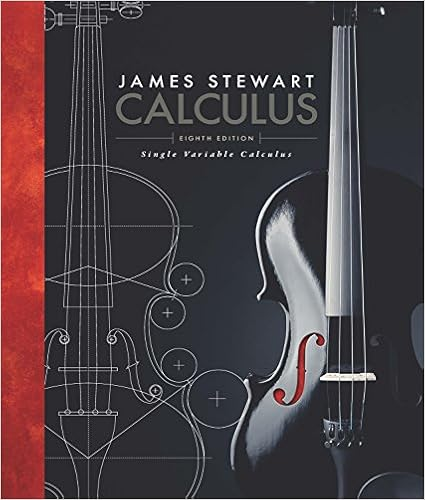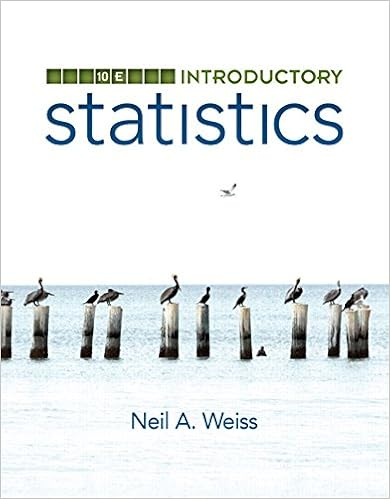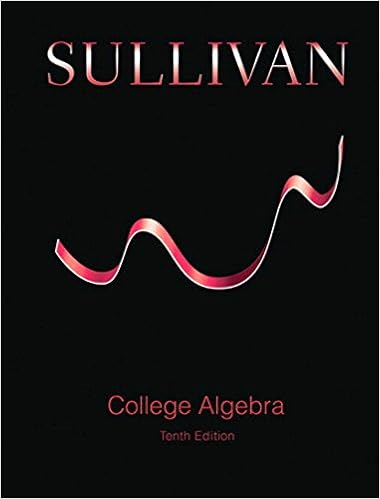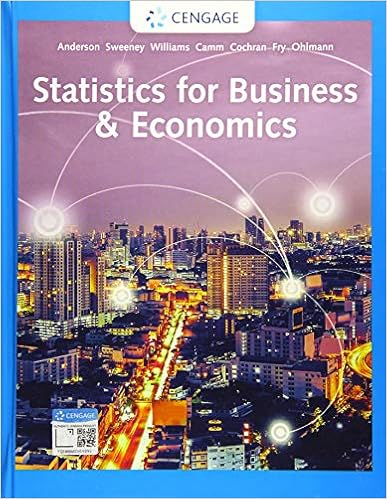# note11.pdf - 2017-18 First Semester MATH 3600 Discrete...

• Notes
• 15
• 100% (1) 1 out of 1 people found this document helpful

This preview shows page 1 - 4 out of 15 pages.

12017-18First SemesterMATH 3600 Discrete MathematicsChapter 11:Introduction to Graph TheoryCoverage of Chapter 11:Sections 11.1 to 11.5 will be covered.A.Terminology and Basic Properties(Ref: Section 11.1)1.(a)When did you first learnof the term ‘graph’ in mathematics?(b)What are the ‘graphs’ in graph theory?(c)Think of daily life examples in which representation by ‘graphs’ will be useful.2.Formally, agraphGis an ordered pair (V,E(a)Vis the set of v________ and its cardinality is known as the o______ ofG(b)Eis the set of e_____ and each element ofEis of the form ______ where,x yV(c) IfE, there are different ways of describing its two elements (say,xandy), such as:xandyareadjacentxandyare i__________ tojoinsxandxandyare n____________)...y
##### We have textbook solutions for you!The document you are viewing contains questions related to this textbook.
Chapter 1 / Exercise 23
Single Variable Calculus
StewartExpert VerifiedBrowse all Textbook Solutions
3.The ‘graph’ defined in the previously question is sometimes known as asimple graph(a)Two generalisations of a simple graph are as follows.(i)If we allowmultiple edges, then such a graph will be called amultigraph. Wesometimes use{ , }m x yto denote themultiplicityof the edge{ , }x y(ii) If we further allowloops, then the resulting graph will be called ageneral graph(b)Can you think of daily life scenarios in which such representations will be useful?...
4.Some common types of graphs areK,C
2
35.(a)Draw4Kin two ‘different’ ways.(b)The two graphs in (a), although ‘different’, are actually ‘the same’. Explain what wemean by saying that two graphs areisomorphic6.Thedegreeof a vertexx, denoteddeg( )x, is the number of edges incident to(a)Why should we pay attention when discussing degrees if loops are present?(b)What is the sum of the degrees of all vertices in a graph?(c)What is meant by thedegree sequenceof a graph?(d) We say that a graph isk-regularif ___________________________,regularif it isregular for somek(Note: Somehow confusingly, a 0-regular graph is said to be anull graphor anemptygraph, while both terms are sometimes used to denote a graph whose vertex set is empty.).x.k-.

Upload your study docs or become a

Course Hero member to access this document

Upload your study docs or become a

Course Hero member to access this document

End of preview. Want to read all 15 pages?

Upload your study docs or become a

Course Hero member to access this document

Term
Fall
Professor
law
Tags
Math,
##### We have textbook solutions for you!
The document you are viewing contains questions related to this textbook.The document you are viewing contains questions related to this textbook.
Chapter 1 / Exercise 23
Single Variable Calculus
StewartExpert Verified
•••